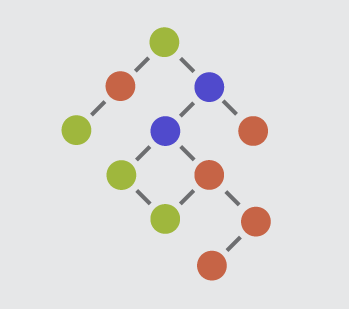# Leaves On A Binary Search TreeThe following python code describes an algorithm for inserting values into a binary search tree.

  1 2 3 4 5 6 7 8 9 10 11 12 13 14 15 16 17 18 19 class Node: def __init__(self, val): self.left_child = None self.right_child = None self.data = val def binary_insert(tree, val): # insert val at root if tree is empty if tree is None: tree = Node(val) else: # if val is less than current node, # insert val into left subtree if (tree.data > val): tree.left_child = binary_insert(tree.left_child, val) # else, insert val into right subtree else: tree.right_child = binary_insert(tree.right_child, val) return tree 

Using this algorithm, you create a new binary search tree B, and insert the following elements into it in this order:

 1 4, 2, 1, 5, 6, 3, 7, 9, 8, 12, 10, 11, 13, 15, 14 

After these operations, what is the sum of the data in the leaf nodes of B?

Details and assumptions

A leaf node is a node that has no children (no left_child or right_child).

×